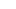# FREE Printable Interactive Multiplication Mats for Teaching Kids to Multiply

When you first begin to teaching multiplication, it’s important to focus on teaching for understanding rather than memorization. While it might be tempting to just jump to learning the facts so you can move on, that’s not going to be helpful in the long run. Instead, focus on the meaning of multiplication, multiple representations, and lots of visuals. These Interactive Multiplication Mats are a great way to begin that process! These math multiplication worksheets help students visualize what is happening when you multiply. These printable multiplication worksheets are perfect for 2nd grade, 3rd grade, and 4th graders. Simply print multiplication worksheets pdf and you are ready to play and learn to multiply!## Multiplication mats

This hands on, free multiplication printable is a fun and handy way to teaching multiplication. Use these multiplication mats to help the concept of multiplying make sense with grade 2, grade 3, and grade 4 students. Whether you are a parent, teacher, or homeschooler looking for printable multiplication worksheets, you will love these handy, FREE, math multiplication worsheets for practicing.  Laminate the math mats to use in your classroom as a math center, help your child get extra practice at home, or use them with your homeschool math curriculum as an easy way to learn multiplication.

## Multiplication worksheets pdf

Start by scrolling to the bottom of the post, under the terms of use, and click on the text link that says >> _____ <<. The pdf file will open in a new window for you to save the freebie template.

## Teaching Multiplication

There are lots of ways to introduce your kids to multiplication and give them a solid foundation for learning. If you start by giving them a list of facts to memorize, it seems like nonsense to them. Consider, for example, if I asked you to remember these:

• A x C = Q
• B x T = Z
• S x U = R

You can look at that and immediately think, “What? That doesn’t make any sense!” But unfortunately, if we try to force kids to memorize multiplication facts, it looks just as nonsensical to them.

Multiplication is not a random set of facts or rules. It’s a logical mathematical computation that has a perfectly reasonable meaning. To help your kids see that, you can start by connecting it to something they know: repeated addition. Then you can show them helpful visuals such as arrays and area models, and how to solve problems with number lines or a hundreds chart.

The more exposure and visual models they have, the better developed their understanding will be, and then eventually “memorized facts” will come.

## Printable multiplication worksheets

Before you begin using these Interactive Multiplication Mats, you will need to print all the mats and laminate them. You can then cut them out and grab some dry erase markers and you’re ready to begin.## Math Multiplication Worksheets

While it is certainly helpful to provide opportunities for kids to practice their multiplication facts, it does not mean that it needs to be drill, flash cards or timed tests.

Instead, find ways to provide “productive practice,” meaning there might be fewer problems to solve, but the challenge is greater and deeper.

There are lots of ways to involve kids in productive practice, and today I’m going to share a resource I created to help kids make sense of multiplication using arrays and area models.## How to Use the Multiplication Equation Mats:

There are 2 sets of multiplication mats in this set (20 mats altogether).

The first set includes a visual model and students have to then write a multiplication equation to match the given model. This is incredibly important, because we all need visuals to form connections in our brain and really learn and internalize mathematical ideas.

This will also help kids understand that a multiplication sentence isn’t meaningless. It’s a numerical representation of the picture.

Once kids are familiar and comfortable with this, they can work on the second set of multiplication mats: the build an equation mats.## Easy Way to Learn Multiplication

The second set reverses the challenge. This time, a multiplication problem is given, and students have to “build” it in some way in order to solve.
This can be done a variety of ways, depending on your kids and what materials you have on hand.
For a hands-on option, we used base ten blocks. But you could also use pom-poms, beads, playdough, etc. Or kids can simply draw a representation using their dry erase marker.## Easy way to teach multiplication

I highly recommend you require students to build or draw the problems, though, before moving on. Even if your child already “knows” the answer, it’s important that they can connect the problem to an accurate visual model. This will help build a foundation for larger multiplication problems in the future, as well as in Algebra.
For more fun, free and LOW PREP math practice, don’t miss our 2000+ page of free math worksheets

## Multiplication Activities## Multiplication for Beginners Worksheets

Before you grab your free pack you agree to the following:

• This set is for personal and classroom use only.
• This printable set may not be sold, hosted, reproduced, or stored on any other website or electronic retrieval system.
• Graphics Purchased and used with permission
• All material provided on this blog is copyright protected.

### >> Interactive Multiplication Mats <<